# Texas Go Math Grade 5 Lesson 12.3 Answer Key Volume of Rectangular Prisms

Refer to our Texas Go Math Grade 5 Answer Key Pdf to score good marks in the exams. Test yourself by practicing the problems from Texas Go Math Grade 5 Lesson 12.3 Answer Key Volume of Rectangular Prisms.

## Texas Go Math Grade 5 Lesson 12.3 Answer Key Volume of Rectangular Prisms

Essential Question
How can you find the volume of a rectangular prism?
To find the volume of a rectangular prism, multiply its 3 dimensions: length x width x height. The volume is expressed in cubic units.

Connect The base of a rectangular prism is a rectangle. You know that area is measured in square units and that the area of a rectangle can be found by multiplying the length and the width.
Volume is measured in cubic units, such as cubic feet or cu ft. When you build a prism and add each layer of cubes, you are adding a third dimension, height.Unlock the Problem

Sid built the rectangular prism shown at the right, using 1-inch cubes. The prism has a base that is a rectangle and has a height of 4 cubes. What is the volume of the rectangular prism that Sid built?

You can find the volume of a prism in cubic units by multiplying the number of square units in the base shape by the number of layers, or its height.
Each layer of Sid’s rectangular prism is composed of 12-inch cubes.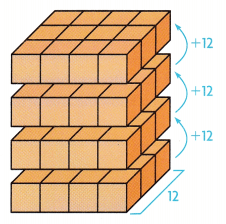Question 1.
How does the volume change as each layer is added?
The given figure is:Now,
From the given figure,
We can observe that
12 cubes are increased to the previous layer of cubes when a new layer is added
Hence, from the above,
We can conclude that
The volume will increase to 12 cubes when we add a new layer

Question 2.
What does the number you multiply the height by represent?
We know that,
The volume of a rectangular prism = Base × Height
Where,
The Base of the rectangular prism is a “rectangle”
So,
The “Base” is the area of the rectangle
So,
Base = Length × Width
Hence, from the above,
We can conclude that
The number you multiply the height by represents the “Volume” of a rectangular prism
So,
The volume of Sid’s rectangular prism is 48 cu in.

Share and Show

Find the volume.

Question 1.
The length of the rectangular prism is ___.
The width is ___. So, the area of the base is ____.
The height is ___. So, the volume of the prism is ___.The given figure is:Now,
From the given figure,
We can observe that
The length of the rectangular prism is: 2 cm
The width of the rectangular prism is: 4 cm
So,
The area of the base = Length × Width
= 2 × 4
= 8 cm²
Now,
The height of the rectangular prism is: 5 cm
So,
The volume of the rectangular prism = Base × Height
= 8 × 5
= 40 cm³
Hence, from the above,
We can conclude that
The volume of the prism is: 40 cm³

Question 2.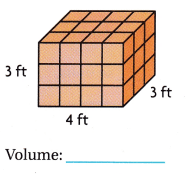The given figure is: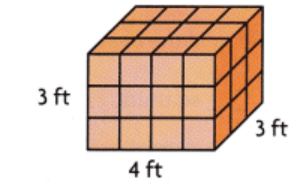Now,
We know that,
The volume of a rectangular prism = Length × Width × Height
So,
The volume of the given figure = 4 × 3 × 3
= 36 ft³
Hence, from the above,
We can conclude that
The volume of the given figure is: 36 ft³

Question 3.The given figure is: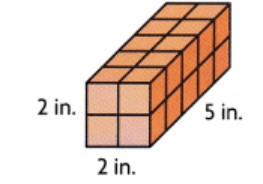Now,
We know that,
The volume of a rectangular prism = Length × Width × Height
So,
The volume of the given figure = 2 × 2 × 5
= 20 ft³
Hence, from the above,
We can conclude that
The volume of the given figure is: 20 ft³

Problem Solving

Question 4.
Explain what else you need to know to find the volume of a rectangular prism if you know the area of the base.
We know that,
The volume of a rectangular prism = Base × Height
Now,
If we know the area of the base, then we have to find the height to find the volume of the rectangular prism
Hence, from the above,
We can conclude that
you need to know the height to find the volume of a rectangular prism if you know the area of the base.

Question 5.
H.O.T. Reasoning How can you find the volume of a cube if you know the area of the base is 25 sq cm?
We know that,
The volume of a rectangular prism = Area of the Base × Height
Now,
If we know the area of the base, then we have also to know the height of the cube to find the volume of the cube
Hence, from the above,
We can conclude that
The volume of a rectangular prism = Area of the Base × Height

Question 6.
H.O.T. The volume of a rectangular prism is 40 cu ft. The height of the prism is 10 ft. What is the area of the base of the prism? Explain.
It is given that
The volume of a rectangular prism is 40 cu ft. The height of the prism is 10 ft
Now,
We know that,
The volume of a rectangular prism = Area of the Base × Height
So,
40 = Area of the Base × 10
Area of the base = $$\frac{40}{10}$$
Area of the Base = 4 ft²
Hence, from the above,
We can conclude that
The area of the base of the prism is 4 ft²

Problem Solving

Question 7.
Kayan is designing a box for sugar cubes. Each sugar cube has a volume of 1 cubic inch. Kayan wants to have 12 cubes in each layer. If he wants to fit 60 cubes in each box, how tall should the box be?
It is given that
Kayan is designing a box for sugar cubes. Each sugar cube has a volume of 1 cubic inch. Kayan wants to have 12 cubes in each layer and he wants to fit 60 cubes in each box
Now,
We know that,
The volume of a rectangular prism = Area of the Base × Height
So,
According to the given information,
60 = 12 × Height
Height = $$\frac{60}{12}$$
Height = 5 inches
Hence, from the above,
We can conclude that
The height of the box should be: 5 inches

Question 8.
H.O.T. Multi-Step Golf balls are packed in cardboard boxes that are 2 inches long, 2 inches wide, and 2 inches high. The boxes are then packed into larger containers that are 20 inches long, 30 inches wide, and 20 inches high. How many golf balls can fit in the larger container?It is given that
Golf balls are packed in cardboard boxes that are 2 inches long, 2 inches wide, and 2 inches high. The boxes are then packed into larger containers that are 20 inches long, 30 inches wide, and 20 inches high
Now,
According to the given information,
The volume of the cardboard boxes = 2 × 2 × 2
= 8 inches³
The volume of the large containers = 20 × 30 × 20
= 12,000 inches³
Now,
The number of golf balls that can fit into the large container = $$\frac{12,000}{8}$$
= 1,500
Hence, from the above,
We can conclude that
The number of golf balls that can fit into the large container is: 1,500 golf balls

Question 9.
H.O.T. Multi-Step Yvette uses centimeter cubes to make a bed for her doll. The bed is in the shape of a rectangular prism. She wants the length of the prism to be 15 cm. The width should be 5 cm and the height 5 cm. How many centimeter cubes does Yvette need?
It is given that
Yvette uses centimeter cubes to make a bed for her doll. The bed is in the shape of a rectangular prism. She wants the length of the prism to be 15 cm. The width should be 5 cm and the height 5 cm
Now,
According to the given information,
The number of centimeter cubes does Yvette needed = Length × Width × Height
= 15 × 5 × 5
= 375 centimeter cubes
Hence, from the above,
We can conclude that
The number of centimeter cubes does Yvette needed is: 375-centimeter cubes

Question 10.
Multi-Step Cameron uses a rectangular baking pan with a base measuring 12 square inches. Cameron pours batter in the pan so that it is 2 inches deep. The finished cake will be 3 times as tall as the batter. What is the volume of the cake?
It is given that
Cameron uses a rectangular baking pan with a base measuring 12 square inches. Cameron pours batter into the pan so that it is 2 inches deep. The finished cake will be 3 times as tall as the batter
So,
The volume of the rectangular baking pan = Area of the Base × height
= 12 × 2
= 24 inches³
So,
The volume of the cake = 3 × (The volume of the rectangular baking pan)
= 3 × 24
= 72 inches³
Hence, from the above,
We can conclude that
The volume of the cake is: 72 inches³

Question 11.
A cube-shaped block of cheese has side lengths of 6 inches. The block of cheese is cut into smaller pieces. Each piece has a volume of 1 cubic inch. How many pieces of cheese will there be?
(A) 18 pieces
(B) 36 pieces
(C) 216 pieces
(D) 72 pieces
It is given that
A cube-shaped block of cheese has side lengths of 6 inches. The block of cheese is cut into smaller pieces. Each piece has a volume of 1 cubic inch
Now,
According to the given information,
The number of pieces of cheese there will be = (The volume of a cube-shaped block of cheese) ÷ (The volume of each piece)
= $$\frac{6 × 6 × 6}{1}$$
= 216 pieces
Hence, from the above,
We can conclude that
The number of pieces of cheese there will be is:Question 12.
Frank is packing cube-shaped containers into large boxes. He can fit 15 containers in each layer. If he stacks 8 layers into one box, what is the volume of the box?
(A) 64 cubes
(B) 120 cubes
(C) 128 cubes
(D) 256 cubes
It is given that
Frank is packing cube-shaped containers into large boxes. He can fit 15 containers in each layer and he stacks 8 layers into one box
Now,
According to the given information,
The volume of the box = (The number of containers in each layer) × (The number of layers)
= 15 × 8
= 120 cubes
Hence, from the above,
We can conclude that
The volume of the box is:Question 13.
Multi-Step A rectangular block of cheese has a length of 8 centimeters, a width of 4 centimeters, and a height of 3 centimeters. If the block is cut into two equal pieces, what will be the volume of each piece?
(A) 15 cubic cm
(B) 28 cubic cm
(C) 48 cubic cm
(D) 96 cubic cm
It is given that
A rectangular block of cheese has a length of 8 centimeters, a width of 4 centimeters, and a height of 3 centimeters, and the block is cut into two equal pieces
Now,
According to the given information,
The volume of the rectangular block of cheese = Length × Width × Height
= 8 × 4 × 3
= 96 cm³
Now,
The volume of each block of cheese when it is divided into 2 pieces = $$\frac{96}{2}$$
= 48 cm³
Hence, from the above,
We can conclude that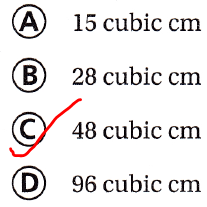Texas Test Prep

Question 14.
What is the volume of the rectangular prism at the right?
(A) 28 sq cm
(B) 140 sq cm
(C) 28 cu cm
(D) 140 cu cmThe given figure is:Now,
The volume of the given figure = Length × Width × Height
= 5 × 7 × 4
= 140 cm³
Hence, from the above,
We can conclude that### Texas Go Math Grade 5 Lesson 12.3 Homework and Practice Answer Key

Find the volume.

Question 1.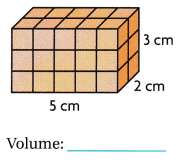The given figure is: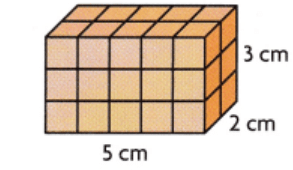Now,
We know that,
Volume = Length × Width × Height
So,
The volume of the given figure = 5 × 3 × 2
= 30 cm³
Hence, from the above,
We can conclude that
The volume of the given figure is: 30 cm³

Question 2.The given figure is:Now,
We know that,
Volume = Length × Width × Height
So,
The volume of the given figure = 8 × 3 × 2
= 48 ft³
Hence, from the above,
We can conclude that
The volume of the given figure is: 48 ft³

Question 3.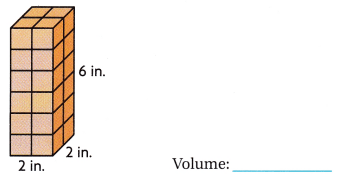The given figure is: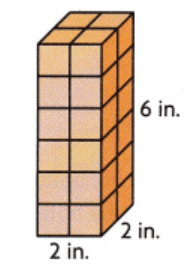Now,
We know that,
Volume = Length × Width × Height
So,
The volume of the given figure = 6 × 2 × 2
= 24 in³
Hence, from the above,
We can conclude that
The volume of the given figure is: 24 in³

Question 4.The given figure is:Now,
We know that,
Volume = Length × Width × Height
So,
The volume of the given figure = 5 × 3 × 3
= 45 cm³
Hence, from the above,
We can conclude that
The volume of the given figure is: 45 cm³

Question 5.The given figure is:Now,
We know that,
Volume = Length × Width × Height
So,
The volume of the given figure = 5 × 3 × 4
= 60 in.³
Hence, from the above,
We can conclude that
The volume of the given figure is 60 in.³

Question 6.The given figure is:Now,
We know that,
Volume = Length × Width × Height
So,
The volume of the given figure = 2 × 3 × 4
= 24 ft³
Hence, from the above,
We can conclude that
The volume of the given figure is: 24 ft³

Problem Solving

Question 7.
Vijay has a collection of 50 number cubes that are each 1 cubic inch. He finds a box that is 4 inches high. He fits 12 cubes into the bottom of the box, Can Vijay fit all his number cubes into the box? Explain.
It is given that
Vijay has a collection of 50 number cubes that are each 1 cubic inch. He finds a box that is 4 inches high. He fits 12 cubes into the bottom of the box
Now,
According to the given information,
The number of cubes present in the box = 4 × 12
= 48 cubes
But,
He has to keep 50 cubes in the box
So,
48 < 50
Hence, from the above,
We can conclude that
Vijay can’t fit all his number cubes into the box

Question 8.
Maya wants to use 1-centimeter cubes to make a larger cube with side lengths of 3 centimeters. How many 1-centimeter cubes will Maya need?
It is given that
Maya wants to use 1-centimeter cubes to make a larger cube with side lengths of 3 centimeters
Now,
According to the given information,
The number of 1-centimeter cubes Maya will need = 3 × 3 × 3
= 27 1-centimeter cubes
Hence, from the above,
We can conclude that
The number of 1-centimeter cubes Maya will need is: 27 1-centimeter cubes

Texas Test Prep

Question 9.
What is the volume of the rectangular prism shown below?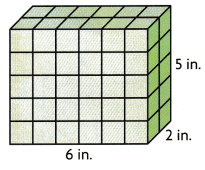(A) 12 sq in.
(B) 60 sq in.
(C) 12 cu in.
(D) 60 cu in.
The given figure is:Now,
We know that,
Volume = Length × Width × Height
So,
The volume of the given figure = 6 × 2 × 5
= 60 in.³
Hence, from the above,
We can conclude thatQuestion 10.
Miranda packs cube-shaped jewelry boxes into a display box that is a rectangular prism. In the bottom layer, she can fit 4 rows of 7 jewelry boxes. If she stacks 6 layers into the display box, what is the volume of the display box?
(A) 168 cubes
(B) 28 cubes
(C) 196 cubes
(D) 66 cubes
It is given that
Miranda packs cube-shaped jewelry boxes into a display box that is a rectangular prism. In the bottom layer, she can fit 4 rows of 7 jewelry boxes. and she stacks 6 layers into the display box
Now,
According to the given information,
The volume of the display box = (The number of rows × The number of jewelry boxes) × (The number of layers)
= 4 × 7 × 6
= 168 cubes
Hence, from the above,
We can conclude that
The volume of the display box is: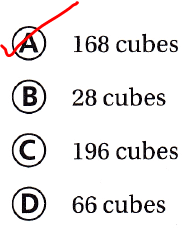Question 11.
Jaden fills a box with 32 cubes. The box is 4 cubes long and 2 cubes wide. How many layers of cubes are in the box?
(A) 3
(B) 4
(C) 8
(D) 5
It is given that
Jaden fills a box with 32 cubes. The box is 4 cubes long and 2 cubes wide
Now,
According to the given information,
The volume of the box = (Base Area) × (Height or the number of layers)
32 = 4 × 2 × The number of layers
The number of layers of cubes in the box = $$\frac{32}{8}$$
= 4 layers
Hence, from the above,
We can conclude that
The number of layers of cubes in the box is:Question 12.
Javier has forty 1-centimeter cubes. He builds a rectangular prism with 6 cubes in the bottom layer. He wants to have the fewest cubes leftover. How many layers can Javier make?
(A) 7
(B) 5
(C) 6
(D) 8
It is given that
Javier has forty 1-centimeter cubes. He builds a rectangular prism with 6 cubes in the bottom layer. He wants to have the fewest cubes leftover
Now,
According to the given information,
The number of layers can Javier make = $$\frac{40}{6}$$
= 6 R 4
Hence, from the above,
We can conclude that
The number of layers can Javier make is: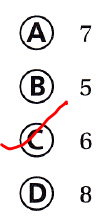Question 13.
Multi-Step The shipping clerk packs a cube with side lengths of 8 inches into a box with side lengths of 10 inches. What is the volume of the space left in the larger box for packing materials?
(A) 598 cubic inches
(B) 1,000 cubic inches
(C) 512 cubic inches
(D) 488 cubic inches
It is given that
The shipping clerk packs a cube with side lengths of 8 inches into a box with side lengths of 10 inches
Now,
According to the given information,
The volume of the space left in the larger box for packing materials = (10 × 10 × 10) – (8 × 8 × 8)
= 1,000 – 512
= 488 in.³
Hence, from the above,
We can conclude that
The volume of the space left in the larger box for packing materials is: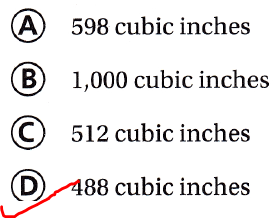Question 14.
Multi-Step The volume of cube A is 64 cubic feet. What is the volume of cube B if its side lengths are half the length of cube A’s sides?
(A) 8 cubic feet
(B) 32 cubic feet
(C) 16 cubic feet
(D) 4 cubic feet
It is given that
The volume of cube A is 64 cubic feet.
So,
V = 64
V = 4³
$$\sqrt{Side³}$$ = $$\sqrt{4³}$$
Side = 4 feet
So,
The side lengths of cube B = $$\frac{4}{2}$$
= 2 feet
So,
The volume of cube B = 2 × 2 × 2
= 8 ft³
Hence, from the above,
We can conclude that
The volume of cube B is: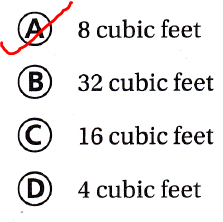Scroll to Top